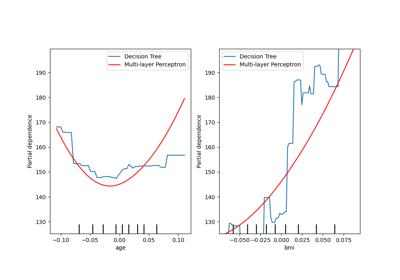# sklearn.inspection.PartialDependenceDisplay¶

class sklearn.inspection.PartialDependenceDisplay(pd_results, *, features, feature_names, target_idx, pdp_lim, deciles)[source]

Partial Dependence Plot (PDP) visualization.

It is recommended to use plot_partial_dependence to create a PartialDependenceDisplay. All parameters are stored as attributes.

Read more in Advanced Plotting With Partial Dependence and the User Guide.

New in version 0.22.

Parameters
pd_resultslist of (ndarray, ndarray)

Results of partial_dependence for features. Each tuple corresponds to a (averaged_predictions, grid).

featureslist of (int,) or list of (int, int)

Indices of features for a given plot. A tuple of one integer will plot a partial dependence curve of one feature. A tuple of two integers will plot a two-way partial dependence curve as a contour plot.

feature_nameslist of str

Feature names corresponding to the indices in features.

target_idxint
• In a multiclass setting, specifies the class for which the PDPs should be computed. Note that for binary classification, the positive class (index 1) is always used.

• In a multioutput setting, specifies the task for which the PDPs should be computed.

Ignored in binary classification or classical regression settings.

pdp_limdict

Global min and max average predictions, such that all plots will have the same scale and y limits. pdp_lim is the global min and max for single partial dependence curves. pdp_lim is the global min and max for two-way partial dependence curves.

decilesdict

Deciles for feature indices in features.

Attributes
bounding_ax_matplotlib Axes or None

If ax is an axes or None, the bounding_ax_ is the axes where the grid of partial dependence plots are drawn. If ax is a list of axes or a numpy array of axes, bounding_ax_ is None.

axes_ndarray of matplotlib Axes

If ax is an axes or None, axes_[i, j] is the axes on the i-th row and j-th column. If ax is a list of axes, axes_[i] is the i-th item in ax. Elements that are None correspond to a nonexisting axes in that position.

lines_ndarray of matplotlib Artists

If ax is an axes or None, lines_[i, j] is the partial dependence curve on the i-th row and j-th column. If ax is a list of axes, lines_[i] is the partial dependence curve corresponding to the i-th item in ax. Elements that are None correspond to a nonexisting axes or an axes that does not include a line plot.

deciles_vlines_ndarray of matplotlib LineCollection

If ax is an axes or None, vlines_[i, j] is the line collection representing the x axis deciles of the i-th row and j-th column. If ax is a list of axes, vlines_[i] corresponds to the i-th item in ax. Elements that are None correspond to a nonexisting axes or an axes that does not include a PDP plot. .. versionadded:: 0.23

deciles_hlines_ndarray of matplotlib LineCollection

If ax is an axes or None, vlines_[i, j] is the line collection representing the y axis deciles of the i-th row and j-th column. If ax is a list of axes, vlines_[i] corresponds to the i-th item in ax. Elements that are None correspond to a nonexisting axes or an axes that does not include a 2-way plot. .. versionadded:: 0.23

contours_ndarray of matplotlib Artists

If ax is an axes or None, contours_[i, j] is the partial dependence plot on the i-th row and j-th column. If ax is a list of axes, contours_[i] is the partial dependence plot corresponding to the i-th item in ax. Elements that are None correspond to a nonexisting axes or an axes that does not include a contour plot.

figure_matplotlib Figure

Figure containing partial dependence plots.

Methods

 plot([ax, n_cols, line_kw, contour_kw]) Plot partial dependence plots.
__init__(pd_results, *, features, feature_names, target_idx, pdp_lim, deciles)[source]

Initialize self. See help(type(self)) for accurate signature.

plot(ax=None, n_cols=3, line_kw=None, contour_kw=None)[source]

Plot partial dependence plots.

Parameters
axMatplotlib axes or array-like of Matplotlib axes, default=None
• If a single axis is passed in, it is treated as a bounding axes

and a grid of partial dependence plots will be drawn within these bounds. The n_cols parameter controls the number of columns in the grid.

• If an array-like of axes are passed in, the partial dependence

plots will be drawn directly into these axes.

• If None, a figure and a bounding axes is created and treated

as the single axes case.

n_colsint, default=3

The maximum number of columns in the grid plot. Only active when ax is a single axes or None.

line_kwdict, default=None

Dict with keywords passed to the matplotlib.pyplot.plot call. For one-way partial dependence plots.

contour_kwdict, default=None

Dict with keywords passed to the matplotlib.pyplot.contourf call for two-way partial dependence plots.

Returns
display: PartialDependenceDisplay

## Examples using sklearn.inspection.PartialDependenceDisplay¶